# Java: LOL!: Gamification of a DataSET :D

Randomized multiple choice quiz of the relationship sets of Fallout 2 Characters and their Locations.

(Character (is from/is related to) Location)
(Concept 1 -> Relationship -> Concept 2)
Example:
( Sulik from Klamath)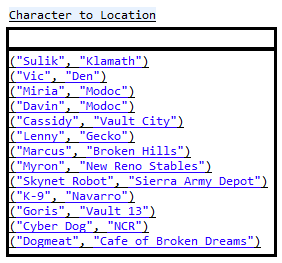Now we want to ask a random question, so we get a random number between 1 and 13 , and pick that row in the data set.

We roll the dice and get “1” so we take the first data row and ask

“Where is Sulik Found?”

This quiz is going to be multiple choice of 4, so we need 3 random incorrect answers, and we need to randomize the correct answer’s location somewhere within the set of incorrect answers.

Make a List of 3 unique/distinct incorrect answers out of the remaining values within the hashtable.  Again, exclude the correct answer  and handle any duplicates.  So in Sulik‘s case, the distinct set of potential incorrect answers looks like this.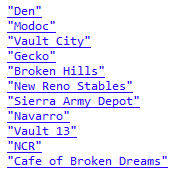Pick another random number and pick 3 distinct values from this list.  Then, append the 3 incorrect values with the correct one.  Pick another random random number 1-4 and display the randomized answers 4 times for “a.)” through “d.)“.

code for now…

```import java.util.Enumeration; import java.util.Hashtable; import java.util.LinkedList; import java.util.ListIterator; import java.util.Random;   public class QuizStructure { public static void main(String[] args) { LinkedList EveryPotentialQuestion_AnswerSet = new LinkedList();   //HOW TO MAKE A Randomized QUIZ off OF a DataSet   //Gamification of a DataSET// LOL :D   System.out.println("indeed."); Hashtable Character_to_Location = new Hashtable();   //"FALLOUT 2 Black Isle Character to Location" Relationship// //( Character - Is_From - Location )// //( Concept - Relationship - Concept ) Character_to_Location.put("Sulik", "Klamath"); Character_to_Location.put("Vic", "Den"); Character_to_Location.put("Miria", "Modoc"); Character_to_Location.put("Davin", "Modoc"); Character_to_Location.put("Cassidy", "Vault City"); Character_to_Location.put("Lenny", "Gecko"); Character_to_Location.put("Marcus", "Broken Hills"); Character_to_Location.put("Myron", "New Reno Stables"); Character_to_Location.put("Skynet Robot", "Sierra Army Depot"); Character_to_Location.put("K-9", "Navarro"); Character_to_Location.put("Goris", "Vault 13"); Character_to_Location.put("Cyber Dog", "NCR"); Character_to_Location.put("Dogmeat", "Cafe of Broken Dreams"); System.out.println(Character_to_Location.toString()); Enumeration keys = Character_to_Location.keys(); while( keys.hasMoreElements() ) { Object key = keys.nextElement(); Object value = Character_to_Location.get(key); System.out.println("Question/Answer Preview: Where is "+key+" from in Fallout 2? Answer : "+value); } Random randomGenerator = new Random(); System.out.println("size: "+Character_to_Location.size()); System.out.println("___________________________________"); //ask Ten randomized questions with 4 randomized answers for( int i = 0 ; i &lt; 10 ; i++) { int randomInt = randomGenerator.nextInt(Character_to_Location.size()); randomInt++; int iStop = 0; Enumeration morekeys = Character_to_Location.keys();   while( morekeys.hasMoreElements() ) { Object key = morekeys.nextElement(); Object value = Character_to_Location.get(key); if ( iStop == randomInt) { //System.out.println("index:" + iStop + " "+randomInt); System.out.println(); System.out.println("Where is "+key+" from?"); //Generate Multiple Choice //we know the correct answer is System.out.println(" Correct Answer : " + value); //now we need to generate other "incorrect" Choices //we need 3, Multiple Choice is Typically 4 questions LinkedList wrongAnswerList = new LinkedList(); //while wrongAnswerList is not unique //we want to remove the possibility of duplicates //we want to not include correct answer boolean is_wronglist_unique = false;   while(!is_wronglist_unique) { int randomWrong = randomGenerator.nextInt(Character_to_Location.size()); randomWrong++; Enumeration wrongkeys = Character_to_Location.keys(); int iStopInner =0; while( wrongkeys.hasMoreElements() ) { Object key2 = wrongkeys.nextElement(); Object value2 = Character_to_Location.get(key2); if ( iStopInner == randomWrong) { if ( !wrongAnswerList.contains(value2)) { if(!value2.equals(value)) wrongAnswerList.add(value2); }   } iStopInner++; } if (wrongAnswerList.size() ==3) {is_wronglist_unique = true;} } System.out.println(" Incorrect Answers : "+wrongAnswerList.toString()); System.out.println(); wrongAnswerList.add(value); System.out.println(); String [] AnswerSet = (String[]) wrongAnswerList.toArray(new String); //get 4 random ints :D //0-3 LinkedList numberset = new LinkedList(); boolean isnumbersetunique = false; int deerpCount = 0; while(!isnumbersetunique) { int randomfinal4 = randomGenerator.nextInt(4); if (!numberset.contains(randomfinal4)) { numberset.add(randomfinal4); deerpCount++; } if(deerpCount == 4) { isnumbersetunique = true; String individualquestion =""; System.out.println("** FINAL QUESTION *** Where is "+key+" from?"); individualquestion += "** FINAL QUESTION *** Where is "+key+" from?"; ListIterator itr = numberset.listIterator(); String[] abcd = {"a.)","b.)","c.)","d.)"}; int ix = 0; while(itr.hasNext()) { int get =(int) itr.next(); System.out.println(" " +abcd[ix]+" "+ AnswerSet[get]); individualquestion += " " +abcd[ix]+" "+ AnswerSet[get]; ix++; }   if ( !EveryPotentialQuestion_AnswerSet.contains(individualquestion)) { EveryPotentialQuestion_AnswerSet.add(individualquestion); //how do I know when this ^ is full ?????? ??????? //What is the most optimal way to answer this ^ without doing it manually? } } } wrongAnswerList.clear(); } iStop++; } } //the more questions you ask the more answers you'll get } }```

Output Example: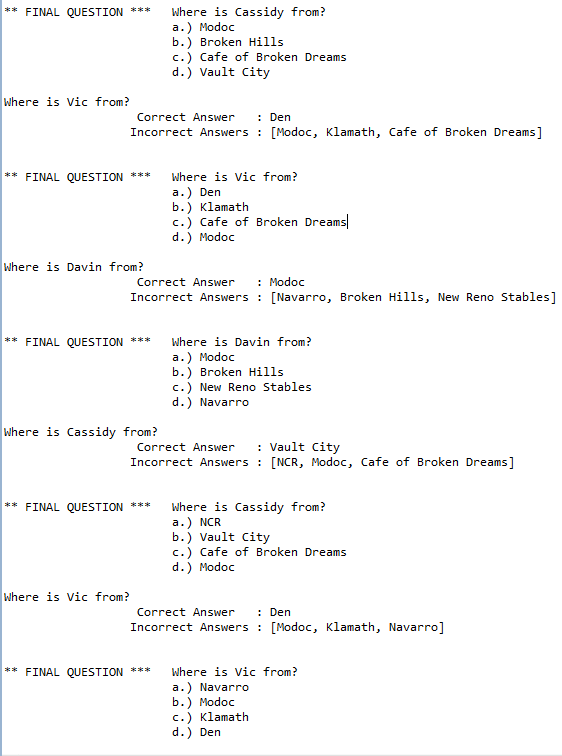What is the total number of distinct Questions and Possible combinations of Answers?  How many unique questions could be asked? Submit your answers below :D

# LOL! Big Data Analytics!

So what’s the best way to compare data?

Say you have a bunch of different data, of all different types and sizes.
You’ve got Square Data and Circle Data.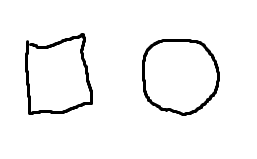And Square Data is Different from Circle Data.
You know they are both Shapes, but one has 4 corners and the other has 0 corners.
“and thats ridiculous!” from either perspective.

Also, if we look closer we can see that circle data looks like this.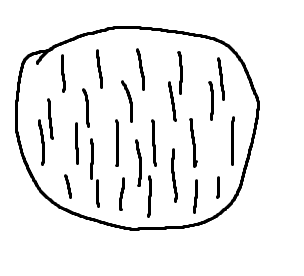And Square Data looks like this.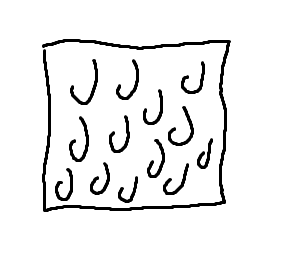Well, I can’t compare this “J” with “l” ! >:O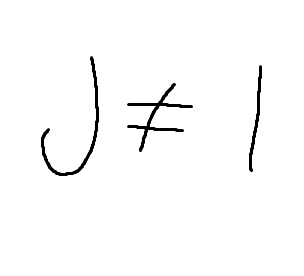WHAT AN OUTRAGE!  This is very frustrating! >:<

. . .

But WHAT IF!  I had even more granular data about each J and l?

And ‘J’ looked like this.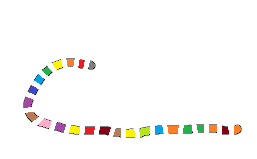And  ‘l’ looked like this.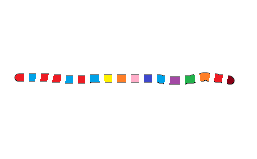:D!   Fantastic!  They have something in common!  COLORS !

“boring!”

meh, so now what? Well, we can record the frequency of each color related to each individual ‘J’ or ‘l’.

“so what?”

And then we can sort the color data of each J or l from most frequent to least.

“lame…”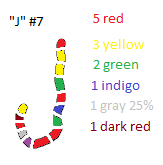“._.”

Then we can take the top x most frequent colors and call that a set (set of colors for each J and l).  So now, by putting emphasis on frequency we can attempt to make relevance!

“Whatever!”

We can now play with Data!

We can figure out and see what the most optimal algorithm for DataSet Comparison is!  Is it top 5 most frequent terms of “J” compared with the top 5 most frequent terms of “l” with at least 2 matches make a relation between “J” and “l”? Maybe its top 15 compared with the top 50 with x matches?  Maybe 5 vs. 15 with x matches?

If the colors were “special” words I find that the  top 10 vs. top 10 with 4 matches or more, works best.  But this could change at any moment!  I could wake up tomorrow and decide differently.  There is no absolute truth here,  teh absolute truth is in teh data!

:D